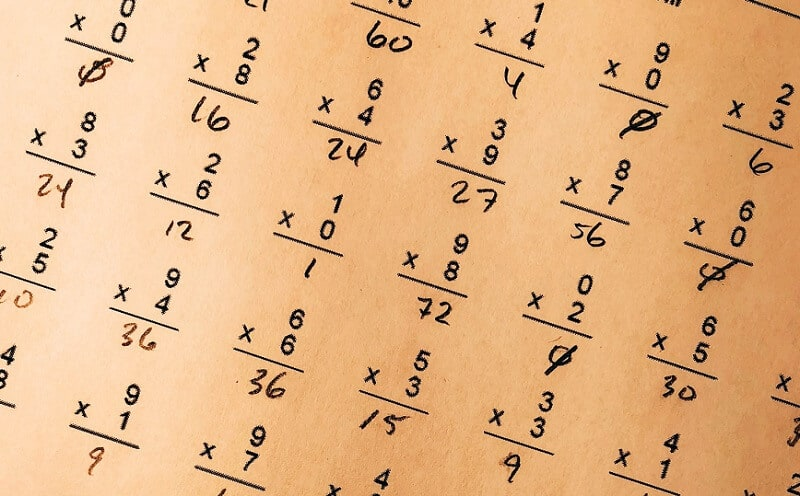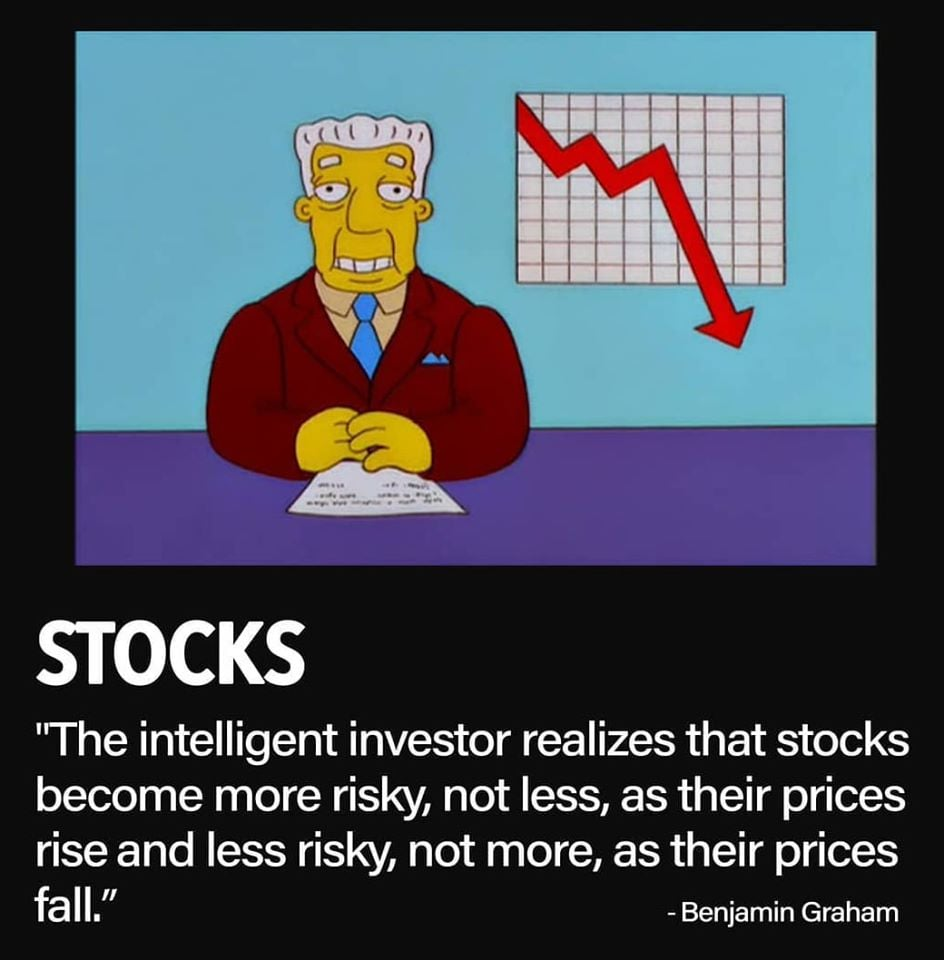## Fair value calculations are essential to any stock investorFair value points to the genuine value of a stock or other security that is agreed between the two parties, the seller and the buyer. It can be calculated for the assets that are traded, but not for the products that are being liquidated. It can be a challenge to calculate the fair value if there are no obviously visible market prices. The point of this is to define the price or value that is fair for both sides, the seller will not be on the losing side, and the buyer will end with a satisfying price.

For example, a trader Anna sells its stocks to the trader John at \$50 per share. The trader John believes he could sell it at \$70 per share once he gets them. So, John buys 1,000 shares at the price Anna is setting. It is a fair value because both sides agreed the price and the trade is beneficial for each of them.### How to calculate fair value?

You can do it with comparable information, for example.

Use respectable financial news and find the last closing price for the stock you want to buy. Say, you want to buy 100 shares of some company and the last closing price of their stocks was \$30. The fair value of 100 shares would be 100 x 30 = \$3,000.

#### Also, you can calculate the fair value using the discounted cash flows.

For example, you want to examine investment that offers a range of cash flows. And you can’t find anything comparable. So, how to calculate the fair value of the investment?

Let’s say it is an investment of \$10,000 and it generates \$2,500 cash flow per year. What you have to do is to write down the cash flows for, let’s say, 5 years.

#### \$10,000

1 – \$2,500
2 – \$2,500
3 – \$2,500
4 – \$2,500
5 – \$2,500

Assume that the rate of return is 6%, and first calculate the discount factor so that you could calculate the discounted cash flows for each year.

For calculation purposes, the percentages need to be transformed into whole numbers. It is done by adding 100 to the number of percents and then dividing that sum by 100, i.e. (100+6)/100=1.06. Now you can calculate the discount factor for each year by rising to power of that year this number, i.e. to the power of 1 for 1st, to power of 2 for 2nd, and so on.

That should look like this:

1.06^1 = 1.06
1.06^2 = 1.12
1.06^3 = 1.19
1.06^4 = 1.26
1.06^5 = 1.34

The next step is to divide every \$2,500 cash flow by the discount factor for each of these five years.

\$2,500 / 1,06 = \$2,359
\$2,500 / 1,12 = \$2,232
\$2,500 / 1.18 = \$2,100
\$2,500 / 1.26 = \$1,984
\$2,500 / 1.34 = \$1,866

This produces five discounted cash flows of:  \$2,359, \$2,232, \$2,119, \$1,984, and \$1,866.
Add these five numbers to -10,000. That was the initial investment, do you remember? Let’s see the result.  The result is 541. This means that using a 6% rate of interest, the fair value of this particular stocks is \$541.

#### Also, you can calculate the fair value for a stock is by using the P/E (price to earnings) ratio.

The formula to calculate the P/E ratio is

the current stock price per share / current earnings per share

What you have to do is to compare P/E ratios among companies from the same industry. For example, if you want to find the fair value for a utility, you have to compare the P/E ratio to other P/E ratios in that industry.

If the company has a high P/E ratio it usually means the company is overvalued. On the other hand, a low P/E ratio shows the company is undervalued. For example, if you hold a stake of shares in a company with a P/E ratio of 4 and the average P/E ratio for other companies in the same industry is 2, you can be sure that your stock is expensive or, in other words, overvalued.

The next thing to do is to modify the stock price to the average P/E ratio. Let’s say the average P/E ratio is 2, and the P/E ratio on your stock is 4. This means the current price is \$8 and earnings per share is \$2. We know that by following the P/E ratio formula.

Use the P/E calculation to find what the stock price needs to have a P/E ratio of 2.

The equation is

#### New P/E ratio x Earnings per share

And the answer is 2 x \$2 or \$4. The fair value for this stock is \$4, not \$8.

#### Bottom line

The puzzle of what security is really worth is one of the basic questions in investing. By calculating fair value, you will find the answer, maybe not exactly but you will be very close to it. Although, fair value calculations are essential to any investor’s stock.

Ways to fair value can classify value investors and growth investors.

The growth investors will estimate earnings that can be unstable. On the other hand, value investors will buy stocks at a discount to their fair value. They will wait for the fair value of their investments to rise.  But both kinds of investors have to know that their companies can stumble. Also, the company may get significantly bigger which will cause keeping historical growth rates difficult.

The point is to buy stocks that will rise to meet the fair value of the company.

#### When unsure what's the right move, you can always trade Forex

##### Get the number #1 winning technical analysis strategy for trading Forex to your email.
Containing the full system rules and unique cash-making strategies. You'll be surprised to see what indicators are being used and what is the master tuning for successful trades. Including case-studies and images.